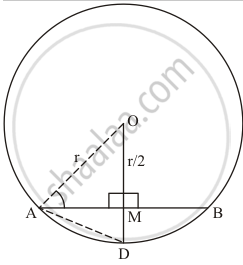Advertisement Remove all ads

# If O is the centre of a circle of radius r and AB is a chord of the circle at a distance r/2 from O, then ∠BAO = - Mathematics

MCQ

If O is the centre of a circle of radius r and AB is a chord of the circle at a distance r/2 from O, then ∠BAO =

#### Options

•  60°

• 45°

•  30°

•  15°

Advertisement Remove all ads

#### Solution

We will associate the given information in the following figure.Since AO = r (radius of circle)

AM = r/2 (given)

Extended OM to D where MD = r/2

Consider the triangles AOM and triangle AMD

OM = MD

angleAMO = angle AMD = 90°

AM = AM    (common Sides

So by SSS property

Δ AMO  ≅ Δ DM

So AD = AO = r and OD=OM+MD=r

Hence ΔAOD is equilateral triangle

So angle OAD = 60°

We know that in equilateral triangle altitudes divide the vertex angles

Therefore           angleOAM = (angleOAD)/2

=60/2

= 30°

Is there an error in this question or solution?
Advertisement Remove all ads

#### APPEARS IN

RD Sharma Mathematics for Class 9
Chapter 15 Circles
Q 3 | Page 110
Advertisement Remove all ads
Advertisement Remove all ads
Share
Notifications

View all notifications

Forgot password?
Course